## Example Questions

### Example Question #3 : Decimal Operations

find 0.72

0.0049

49

4.9

0.49

0.049

0.49

Explanation:

0.7 * 0.7 = 0.49

Trick: do the numbers without the decimals (7*7)

49; move the decimal of the answer the total number of spaces per each number (one for each 0.7)

0.49

### Example Question #1 : How To Multiply Decimals

There are 3,500 people in group A and 5,000 people in group B:

 Car Type % in Group A Who Own % in Group B Who Own Motorbike 4 9 Sedan 35 25 Minivan 22 15 Van 9 12 Coupe 3 6

The number of people in group B who own a minivan is how much greater or less than the number of people in group A who own a minivan?

30 fewer

30 more

20 fewer

50 fewer

15 more

20 fewer

Explanation:

In this question, simply take the percentage of each group that owns a minivan and compare the values. For minivans we look at the middle row which we recognize as a percent that must be converted to a decimal. Acknowledge that there's a different number of people between the groups A and B:

Group B: 0.15(5000) = 750

Group A: 0.22(3500) = 770

750 – 770 = –20; thus, group B has 20 fewer people who own minivans than group A.

### Example Question #2 : How To Multiply Decimals

Given that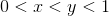, which best describes the relationship between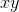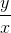, and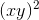?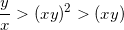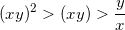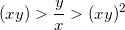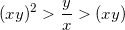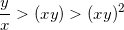Explanation:

We must recognize that if x and y are both between 0 and 1, then they must be fractions (decimals). To determine their relationships, we can substitute values for x and y. Let's have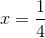and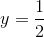(because y must be greater than x).

If x and y are both between 0 and 1, then xy is also between 0 and 1 (though smaller than either x or y individually).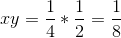The value of (xy)2 will be less than (xy), since squaring any number between 0 and 1 gives a smaller result.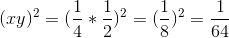Finally,is largest, since dividing a larger number by a smaller one gives a result greater than 1.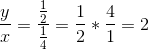Note that the substituted values for x and y were purely hypothetical; any values that satisfy the given inequality could be used.

### Example Question #11 : Decimals

You bought a dozen eggs marked at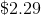and received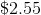change from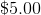. What is the percent of sales tax?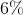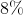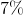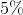Explanation:

Set the equation up as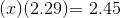Solve for, which equals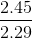or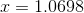Therefore the percent sales tax is: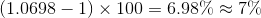Tired of practice problems?

Try live online GRE prep today.